# 12.4 Rotation of axes  (Page 2/8)

 Page 2 / 8

## Identifying a conic from its general form

Identify the graph of each of the following nondegenerate conic sections.

1. $4{x}^{2}-9{y}^{2}+36x+36y-125=0$
2. $9{y}^{2}+16x+36y-10=0$
3. $3{x}^{2}+3{y}^{2}-2x-6y-4=0$
4. $-25{x}^{2}-4{y}^{2}+100x+16y+20=0$
1. Rewriting the general form, we have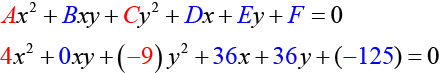$A=4\text{\hspace{0.17em}}$ and $\text{\hspace{0.17em}}C=-9,$ so we observe that $\text{\hspace{0.17em}}A\text{\hspace{0.17em}}$ and $\text{\hspace{0.17em}}C\text{\hspace{0.17em}}$ have opposite signs. The graph of this equation is a hyperbola.

2. Rewriting the general form, we have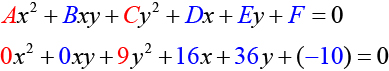$A=0\text{\hspace{0.17em}}$ and $\text{\hspace{0.17em}}C=9.\text{\hspace{0.17em}}$ We can determine that the equation is a parabola, since $\text{\hspace{0.17em}}A\text{\hspace{0.17em}}$ is zero.

3. Rewriting the general form, we have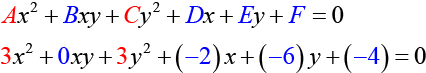$A=3\text{\hspace{0.17em}}$ and $\text{\hspace{0.17em}}C=3.\text{\hspace{0.17em}}$ Because $\text{\hspace{0.17em}}A=C,$ the graph of this equation is a circle.

4. Rewriting the general form, we have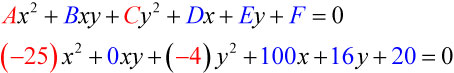$A=-25\text{\hspace{0.17em}}$ and $\text{\hspace{0.17em}}C=-4.\text{\hspace{0.17em}}$ Because $\text{\hspace{0.17em}}AC>0\text{\hspace{0.17em}}$ and $\text{\hspace{0.17em}}A\ne C,$ the graph of this equation is an ellipse.

Identify the graph of each of the following nondegenerate conic sections.

1. $16{y}^{2}-{x}^{2}+x-4y-9=0$
2. $16{x}^{2}+4{y}^{2}+16x+49y-81=0$
1. hyperbola
2. ellipse

## Finding a new representation of the given equation after rotating through a given angle

Until now, we have looked at equations of conic sections without an $\text{\hspace{0.17em}}xy\text{\hspace{0.17em}}$ term, which aligns the graphs with the x - and y -axes. When we add an $\text{\hspace{0.17em}}xy\text{\hspace{0.17em}}$ term, we are rotating the conic about the origin. If the x - and y -axes are rotated through an angle, say $\text{\hspace{0.17em}}\theta ,$ then every point on the plane may be thought of as having two representations: $\text{\hspace{0.17em}}\left(x,y\right)\text{\hspace{0.17em}}$ on the Cartesian plane with the original x -axis and y -axis, and $\text{\hspace{0.17em}}\left({x}^{\prime },{y}^{\prime }\right)\text{\hspace{0.17em}}$ on the new plane defined by the new, rotated axes, called the x' -axis and y' -axis. See [link] .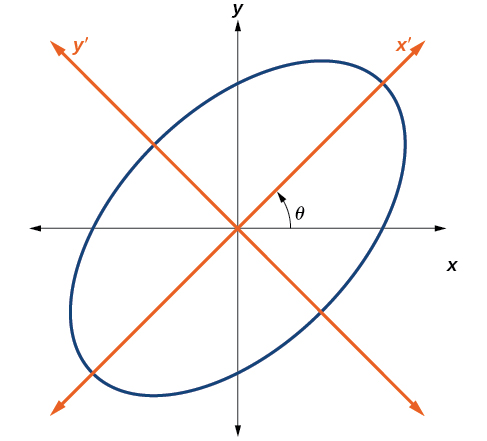The graph of the rotated ellipse   x 2 + y 2 – x y – 15 = 0

We will find the relationships between $\text{\hspace{0.17em}}x\text{\hspace{0.17em}}$ and $\text{\hspace{0.17em}}y\text{\hspace{0.17em}}$ on the Cartesian plane with $\text{\hspace{0.17em}}{x}^{\prime }\text{\hspace{0.17em}}$ and $\text{\hspace{0.17em}}{y}^{\prime }\text{\hspace{0.17em}}$ on the new rotated plane. See [link] .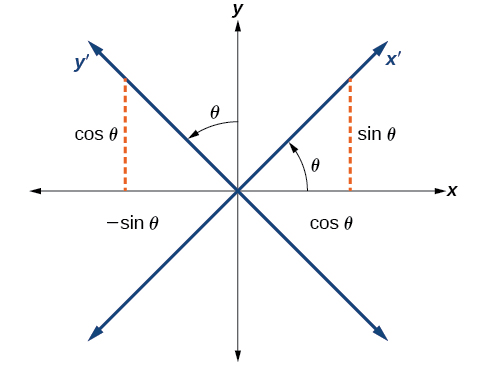The Cartesian plane with x - and y -axes and the resulting x ′− and y ′−axes formed by a rotation by an angle   θ .

The original coordinate x - and y -axes have unit vectors $\text{\hspace{0.17em}}i\text{\hspace{0.17em}}$ and $\text{\hspace{0.17em}}j\text{\hspace{0.17em}}.$ The rotated coordinate axes have unit vectors $\text{\hspace{0.17em}}{i}^{\prime }\text{\hspace{0.17em}}$ and $\text{\hspace{0.17em}}{j}^{\prime }.$ The angle $\text{\hspace{0.17em}}\theta \text{\hspace{0.17em}}$ is known as the angle of rotation    . See [link] . We may write the new unit vectors in terms of the original ones.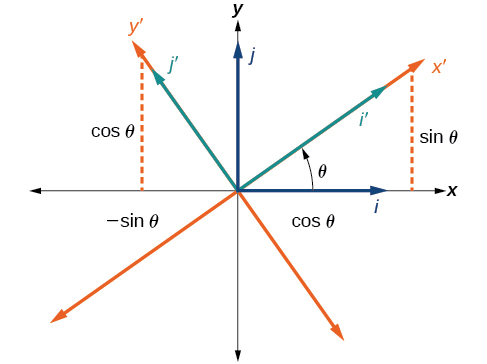Relationship between the old and new coordinate planes.

Consider a vector $\text{\hspace{0.17em}}u\text{\hspace{0.17em}}$ in the new coordinate plane. It may be represented in terms of its coordinate axes.

Because $\text{\hspace{0.17em}}u={x}^{\prime }{i}^{\prime }+{y}^{\prime }{j}^{\prime },$ we have representations of $\text{\hspace{0.17em}}x\text{\hspace{0.17em}}$ and $\text{\hspace{0.17em}}y\text{\hspace{0.17em}}$ in terms of the new coordinate system.

## Equations of rotation

If a point $\text{\hspace{0.17em}}\left(x,y\right)\text{\hspace{0.17em}}$ on the Cartesian plane is represented on a new coordinate plane where the axes of rotation are formed by rotating an angle $\text{\hspace{0.17em}}\theta \text{\hspace{0.17em}}$ from the positive x -axis, then the coordinates of the point with respect to the new axes are $\text{\hspace{0.17em}}\left({x}^{\prime },{y}^{\prime }\right).\text{\hspace{0.17em}}$ We can use the following equations of rotation to define the relationship between $\text{\hspace{0.17em}}\left(x,y\right)\text{\hspace{0.17em}}$ and $\text{\hspace{0.17em}}\left({x}^{\prime },{y}^{\prime }\right):$

and

Given the equation of a conic, find a new representation after rotating through an angle.

1. Find $\text{\hspace{0.17em}}x\text{\hspace{0.17em}}$ and $\text{\hspace{0.17em}}y\text{\hspace{0.17em}}$ where and
2. Substitute the expression for $\text{\hspace{0.17em}}x\text{\hspace{0.17em}}$ and $\text{\hspace{0.17em}}y\text{\hspace{0.17em}}$ into in the given equation, then simplify.
3. Write the equations with $\text{\hspace{0.17em}}{x}^{\prime }\text{\hspace{0.17em}}$ and $\text{\hspace{0.17em}}{y}^{\prime }\text{\hspace{0.17em}}$ in standard form.

#### Questions & Answers

x exposant 4 + 4 x exposant 3 + 8 exposant 2 + 4 x + 1 = 0
x exposent4+4x exposent3+8x exposent2+4x+1=0
HERVE
How can I solve for a domain and a codomains in a given function?
ranges
EDWIN
Thank you I mean range sir.
Oliver
proof for set theory
don't you know?
Inkoom
find to nearest one decimal place of centimeter the length of an arc of circle of radius length 12.5cm and subtending of centeral angle 1.6rad
factoring polynomial
find general solution of the Tanx=-1/root3,secx=2/root3
find general solution of the following equation
Nani
the value of 2 sin square 60 Cos 60
0.75
Lynne
0.75
Inkoom
when can I use sin, cos tan in a giving question
depending on the question
Nicholas
I am a carpenter and I have to cut and assemble a conventional roof line for a new home. The dimensions are: width 30'6" length 40'6". I want a 6 and 12 pitch. The roof is a full hip construction. Give me the L,W and height of rafters for the hip, hip jacks also the length of common jacks.
John
I want to learn the calculations
where can I get indices
I need matrices
Nasasira
hi
Raihany
Hi
Solomon
need help
Raihany
maybe provide us videos
Nasasira
Raihany
Hello
Cromwell
a
Amie
What do you mean by a
Cromwell
nothing. I accidentally press it
Amie
you guys know any app with matrices?
Khay
Ok
Cromwell
Solve the x? x=18+(24-3)=72
x-39=72 x=111
Suraj
Solve the formula for the indicated variable P=b+4a+2c, for b
Need help with this question please
b=-4ac-2c+P
Denisse
b=p-4a-2c
Suddhen
b= p - 4a - 2c
Snr
p=2(2a+C)+b
Suraj
b=p-2(2a+c)
Tapiwa
P=4a+b+2C
COLEMAN
b=P-4a-2c
COLEMAN
like Deadra, show me the step by step order of operation to alive for b
John
A laser rangefinder is locked on a comet approaching Earth. The distance g(x), in kilometers, of the comet after x days, for x in the interval 0 to 30 days, is given by g(x)=250,000csc(π30x). Graph g(x) on the interval [0, 35]. Evaluate g(5)  and interpret the information. What is the minimum distance between the comet and Earth? When does this occur? To which constant in the equation does this correspond? Find and discuss the meaning of any vertical asymptotes.
The sequence is {1,-1,1-1.....} hasByByByByBy David Martin